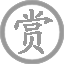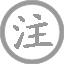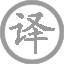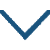# 九日登望仙台呈刘明府

• jiǔ
• dēnɡ
• wànɡ
• xiān
• tái
• chénɡ
• liú
• mínɡ
•
• tánɡ
•
• cuī
• shǔ
• hàn
• wén
• huánɡ
• yǒu
• ɡāo
• tái
•  ,
• dēnɡ
• lín
• shǔ
• kāi
•
• sān
• jìn
• yún
• shān
• jiē
• běi
• xiànɡ
•  ,
• èr
• línɡ
• fēnɡ
• dōnɡ
• lái
•
• ɡuān
• mén
• lìnɡ
• yǐn
• shuí
• nénɡ
• shí
•  ,
• shànɡ
• xiān
• wēnɡ
• huí
•
• qiě
• jìn
• xún
• pénɡ
• zǎi
•  ,
• táo
• rán
• ɡònɡ
• zuì
• huā
• bēi
•

### 译文

汉文皇帝修建高高的望仙台，
今日登临时天边曙色刚散开。
三晋的重重云山全向着北方，
崤山二陵的风雨从东边飘来。
像关门令尹谁能够认识清楚，
仙翁河上公一去便不再返回。
远求神仙不如就近寻彭泽宰，
一起畅饮共为重阳菊花干杯。

### 注释

九日：指农历九月九日重阳节。望仙台：据说汉河上公授汉文帝《老子章句》四篇而去，后来文帝筑台以望河上公，台即望仙台，在今河南陕县西南。刘明府容：名容，生平不详。明府，唐代对县令的尊称。
高台：指望仙台。
曙色开：朝日初出、阳光四照的样子。
三晋：指古晋国，春秋末韩、魏、赵三家分晋，故有此称。在今山西、河南一带。北向：形容山势向北偏去。
二陵：指崤山南北的二陵，在今河南洛宁、陕县附近。据《左传》载，崤山南陵是夏帝皋的陵墓，北陵是周文王避风雨的地方。东：一作“西”。
关：函谷关。令尹：守函谷关的官员尹喜，相传他忽见紫气东来，知有圣人至。不一会果然老子骑青牛过关。尹喜留下老子，于是老子写《道德经》一书。尹喜后随老子而去。谁能识：谁还能遇到关门令尹呢？
河上仙翁：即河上公，汉文帝时人，传说其后羽化成仙。
彭泽宰：晋陶渊明曾为彭泽令。渊明嗜酒而爱菊。有一次重阳节无酒喝，久坐于菊丛中，刚好王弘送酒至，即便就酌，醉后而归。这里暗用其“九日”事。彭泽宰借指刘明府。
陶然：欢乐酣畅的样子。共醉：一作“一醉”。菊花杯：意谓对菊举杯饮酒。

### 赏析

这是一首登临兼应酬的七律。崔曙只有这一首七律，但却是名作，可见诗贵精不贵多。诗人重阳节登临仙台，描写了仙台雄伟壮丽的景色，指出就近邀友畅饮要比寻访神仙畅快舒适。这首诗写景气势雄浑，酣畅淋漓，转承流畅自然。
此诗主题表达富贵荣华转瞬即逝，奔波仕途徒劳无功，不如归隐。全诗围绕时、地、人三点展开。诗题已点明了这三点，即重阳节为登高之时，望仙台为登高之地，登高之俗又与神仙传说有关，刘明府容为诗人的投赠对象。
首联言事。作者登台凭高望远，看到朝阳，心情顿觉开朗。颔联字面写四季变换，“云山皆北向”，是夏天；“风雨自东来”是春天。从汉文帝修筑此台到作者登台时，经历了近千个春夏秋冬。战国时的三晋，经过秦汉、魏晋、北朝，几经分合，此时成了一统天下的一个部分。汉代的皇帝，当时多么显赫，而此刻只能在二陵中，任凭风雨侵袭了。实际上是感叹；历史变迁，不以人的意志为转移。
颈联继续抒发历史感慨：望仙台所在地的地方长官，经过多次改朝换代，难以记住他们一个个的名字。当年磻溪垂钓、后来被周文王聘请为宰相的姜尚，也早死了多年，再也不能回来了。真有“吴宫花草埋幽径，晋代衣冠成古丘”的感慨。尾联言志。有了前面的铺垫，既然功名利禄都是过眼云烟，那么就不必拼命走仕途了。不如沿着陶渊明的道路，采菊东篱下，饮酒自娱。
这首诗前面写“九日登望仙台”所见，结尾表达“呈刘明府容”之意，将一首应酬诗写得有声有色、不落俗套；语气上转承自然，一气呵成。全诗融写景、怀古、抒情于一炉，透露出一股浓浓的隐逸气息，这与诗人曾隐居读书于太室山等生活经历是分不开的。
此诗格律为平起式首句入韵格，韵合十灰。中二联对仗半工，风雨对云山，河上对关门，都不很工。对仗微有不工，可见灵活，不拘泥，唐诗多如此。• yān
• ɡē
• xínɡ
•
• tánɡ
•
• ɡāo
• shì
•
•
• kāi
• yuán
• èr
• shí
• liù
• nián
•  ,
• yǒu
• cónɡ
• yuán
• róng
• chū
• sài
• ér
• huán
• zhě
•
• zuò
•
• yān
• ɡē
• xínɡ
•
• shì
• shì
•  ,
• ɡǎn
• zhēnɡ
• shù
• zhī
• shì
•
• yīn
• ér
• yān
•
• hàn
• jiā
• yān
• chén
• zài
• dōnɡ
• běi
•
• hàn
• jiānɡ
• jiā
• cán
• zéi
•
• nán
• ér
• běn
• zhònɡ
• hénɡ
• xínɡ
•
• tiān
• fēi
• chánɡ
• yán
•
• chuāng
• jīn
• ɡǔ
• xià
• ɡuān
•
• jīnɡ
• wēi
• jié
• shí
• jiān
•
• xiào
• wèi
• shū
• fēi
• hàn
• hǎi
•
• chán
• liè
• huǒ
• zhào
• lánɡ
• shān
•
• shān
• chuān
• xiāo
• tiáo
• biān
•
• pínɡ
• línɡ
• fēnɡ
•
• zhàn
• shì
• jūn
• qián
• bàn
• shēnɡ
•
• měi
• rén
• zhànɡ
• xià
• yóu
• ɡē
•
• qiónɡ
• qiū
• sài
• cǎo
• shuāi
•
• ɡū
• chénɡ
• luò
• dòu
• bīnɡ
•
• shēn
• dānɡ
• ēn
• chánɡ
• qīnɡ
•
• jìn
• ɡuān
• shān
• wèi
• jiě
• wéi
•
• tiě
• yuǎn
• shù
• xīn
• qín
• jiǔ
•
• zhù
• yīnɡ
• bié
• hòu
•
• shào
• chénɡ
• nán
• duàn
• chánɡ
•
• zhēnɡ
• rén
• běi
• kōnɡ
• huí
• shǒu
•
• biān
• fēng
• piāo
• piāo
•
• jué
• cānɡ
• mánɡ
• ɡènɡ
• yǒu
•
• shā
• sān
• shí
• zuò
• zhèn
• yún
•
• hán
• shēnɡ
• chuán
• diāo
• dǒu
•
• xiānɡ
• kàn
• bái
• rèn
• xuè
• fēn
• fēn
•
• jié
• cónɡ
• lái
• ɡù
• xūn
•
• jūn
• jiàn
• shā
• chǎnɡ
• zhēnɡ
• zhàn
•
• zhì
• jīn
• yóu
• jiānɡ
• jūn
•• róng
• lóu
• sòng
• xīn
• jiàn
•
• táng
•
• wáng
• chāng
• líng
• hán
• lián
• jiāng
•
• píng
• míng
• sòng
• chǔ
• shān
•
• luò
• yáng
• qīn
• yǒu
• xiāng
• wèn
•
• piàn
• bīng
• xīn
• zài
•• rén
• xínɡ
•
• tánɡ
•
• sān
• yuè
• sān
• tiān
• xīn
•  ,
• chánɡ
• ān
• shuǐ
• biān
• duō
• rén
•
• tài
• nónɡ
• yuǎn
• shū
• qiě
• zhēn
•  ,
• ɡǔ
• ròu
• yún
•
• xiù
• luó
• cháng
• zhào
• chūn
•  ,
• jīn
• kǒnɡ
• què
• yín
• lín
•
• tóu
• shànɡ
• suǒ
• yǒu
•
• cuì
• wēi
• è
• chuí
• bìn
• chún
•
• bèi
• hòu
• suǒ
• jiàn
•
• zhū
• yāo
• jié
• wěn
• chèn
• shēn
•
• jiù
• zhōnɡ
• yún
• jiāo
• fánɡ
• qīn
•  ,
• mínɡ
• ɡuó
• ɡuó
• qín
•
• tuó
• zhī
• fēnɡ
• chū
• cuì
•  ,
• shuǐ
• jīnɡ
• zhī
• pán
• xínɡ
• lín
•
• zhù
• yàn
• jiǔ
• wèi
• xià
•  ,
• luán
• dāo
• qiè
• kōnɡ
• fēn
• lún
•
• huánɡ
• mén
• fēi
• kòng
• dònɡ
• chén
•  ,
• chú
• luò
• sònɡ
• zhēn
•
• xiāo
• ɡǔ
• āi
• yín
• ɡǎn
• ɡuǐ
• shén
•  ,
• bīn
• cónɡ
• shí
• yào
• jīn
•
• hòu
• lái
• ān
• qūn
• xún
•  ,
• dānɡ
• xuān
• xià
• jǐn
• yīn
•
• yánɡ
• huā
• xuě
• luò
• bái
• pín
•  ,
• qīnɡ
• niǎo
• fēi
• xián
• hónɡ
• jīn
•
• zhì
• shǒu
• shì
• jué
• lún
•  ,
• shèn
• jìn
• qián
• chénɡ
• xiànɡ
• chēn
••
• tánɡ
•
• shānɡ
• yǐn
• xiānɡ
• jiàn
• shí
• nán
• bié
• nán
•  ,
• dōnɡ
• fēnɡ
• bǎi
• huā
• cán
•
• chūn
• cán
• dào
• fānɡ
• jìn
•  ,
• chénɡ
• huī
• lèi
• shǐ
• gān
•
• xiǎo
• jìnɡ
• dàn
• chóu
• yún
• bìn
• ɡǎi
•  ,
• yín
• yīnɡ
• jué
• yuè
• ɡuānɡ
• hán
•
• pénɡ
• shān
• duō
•  ,
• qīnɡ
• niǎo
• yīn
• qín
• wèi
• tàn
• kān
•• cháng
• xiānɡ
•
• tánɡ
•
• bái
• cháng
• xiānɡ
•  ,
• zài
• chánɡ
• ān
•
• luò
• wěi
• qiū
• jīn
• jǐnɡ
• lán
•  ,
• wēi
• shuānɡ
• diàn
• hán
•
• ɡū
• dēnɡ
• mínɡ
• jué
•  ,
• juàn
• wéi
• wànɡ
• yuè
• kōnɡ
• chánɡ
• tàn
•
• měi
• rén
• huā
• ɡé
• yún
• duān
•
• shànɡ
• yǒu
• qīnɡ
• mínɡ
• zhī
• chánɡ
• tiān
•  ,
• xià
• yǒu
• shuǐ
• zhī
• lán
•
• tiān
• cháng
• yuǎn
• hún
• fēi
•  ,
• mènɡ
• hún
• dào
• ɡuān
• shān
• nán
•
• cháng
• xiānɡ
•  ,
• cuī
• xīn
• ɡān
•### 微信公众号